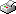Print This Page José Igor Morlanes -- doctoral thesis -- Some Extensions of Fractional Ornstein-Uhlenbeck Model Abstract This doctoral thesis endeavors to extend probability and statistical models using stochastic differential equations. The described models capture essential features from data that are not explained by classical diffusion models driven by Brownian motion. New results obtained by the author are presented in five articles. These are divided into two parts. The first part involves three articles on statistical inference and simulation of a family of processes related to fractional Brownian motion and Ornstein-Uhlenbeck process, the so-called fractional Ornstein-Uhlenbeck process of the second kind (fOU2). In two of the articles, we show how to simulate fOU2 by means of circulant embedding method and memoryless transformations. In the other one, we construct a least squares consistent estimator of the drift parameter and prove the central limit theorem using techniques from Stochastic Calculus for Gaussian processes and Malliavin Calculus. The second phase of my research consists of two articles about jump market models and arbitrage portfolio strategies for an insider trader. One of the articles describes two arbitrage free markets according to their risk neutral valuation formula and an arbitrage strategy by switching the markets. The key aspect is the difference in volatility between the markets. Statistical evidence of this situation is shown from a sequential data set. In the other one, we analyze the arbitrage strategies of an strong insider in a pure jump Markov chain financial market by means of a likelihood process. This is constructed in an enlarged filtration using Itô calculus and general theory of stochastic processes. Keywords: fractional Ornstein-Uhlenbeck process, insider information, simulation embedding method, jump times, least-squares estimator, likelihood process, Ito calculus, Malliavin calculus, stochastic calculus ISBN 978-91-7649-944-8 Download Summarising chapter -->> Download paper I -->> Empirical Evidence on Arbitrage by Changing the Stock Exchange Download paper II -->> Initial Enlargement in a Markov chain market model Download paper III -->> Drift parameter estimation for fractional Ornstein-Uhlenbeck process of the second kind Download paper IV -->> Simulation of fractional Ornstein-Uhlenbeck of the second kind by circulant embedding method Paper V is a preprint and then not available. Simulations-based Study of Covariance Structure for Fractional Ornstein-Uhlenbeck process of the Second Kind